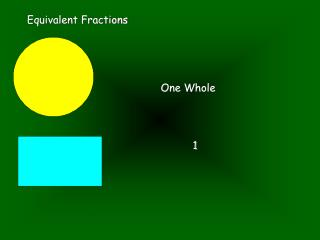DownloadDownload PresentationEquivalent Fractions

# Equivalent Fractions

Télécharger la présentation## Equivalent Fractions

- - - - - - - - - - - - - - - - - - - - - - - - - - - E N D - - - - - - - - - - - - - - - - - - - - - - - - - - -
##### Presentation Transcript

1. Equivalent Fractions One Whole 1

2. Equivalent Fractions Cut them in half

3. Equivalent Fractions Shown is one half 1 2 How many pieces we want How many pieces it’s cut into

4. Equivalent Fractions Shown is one half 1 2 NUMERATOR DENOMINATOR

5. Equivalent Fractions I cut my shape again I still show 1 2

6. Equivalent Fractions But I also show 2 4

7. Equivalent Fractions One half is EQUIVALENT TO 2 quarters 1 2 2 4

8. Equivalent Fractions 1 2 2 4 This symbol looks like an equals sign with a third line. It is the mathematical sign for EQUIVALENT TO - which means “is worth the same as”.

9. Equivalent Fractions We can use equivalent fraction to make our numbers easier to handle. Smaller numbers are SIMPLE 160 200 16 20 4 5 ÷ 10 ÷ 4 ÷ 10 ÷ 4

10. Equivalent Fractions Simplest form Lowest terms Simplified Cancelled down We can use different language for making the fraction as small as possible. Watch out for this language in the future. It is often used in Key Stage 3. 160 200 4 5 This fraction is as simple as we can make it

11. Equivalent Fractions Look for numbers that both the NUMERATOR and the DENOMINATOR can be divided by. We want numbers bigger than 1. 15 45 60 80 We call these COMMON FACTORS

12. Equivalent Fractions These numbers have 3 as a common factor. This means they can both be shared by 3. 15 45 ÷ 3 ÷ 3 60 80 ÷ 10 A common factor here is 10 ÷ 10

13. Equivalent Fractions 15 45 5 15 ÷ 3 ÷ 3 60 80 6 8 ÷ 10 ÷ 10

14. Equivalent Fractions 15 45 5 15 ÷ 3 ÷ 5 ÷ 3 ÷ 5 60 80 6 8 ÷ 10 ÷ 2 ÷ 10 ÷ 2

15. Equivalent Fractions 15 45 5 15 1 3 ÷ 3 ÷ 5 ÷ 3 ÷ 5 60 80 6 8 3 4 ÷ 10 ÷ 2 ÷ 10 ÷ 2

16. Equivalent Fractions If the top number is a 1, we know we can stop. 15 45 1 3 If the top and bottom number are not DIVISIBLE by the same number, we stop. 60 80 3 4

17. Equivalent Fractions They have no FACTORS in common other than 1 15 45 1 3 60 80 3 4 They have no FACTORS in common other than 1